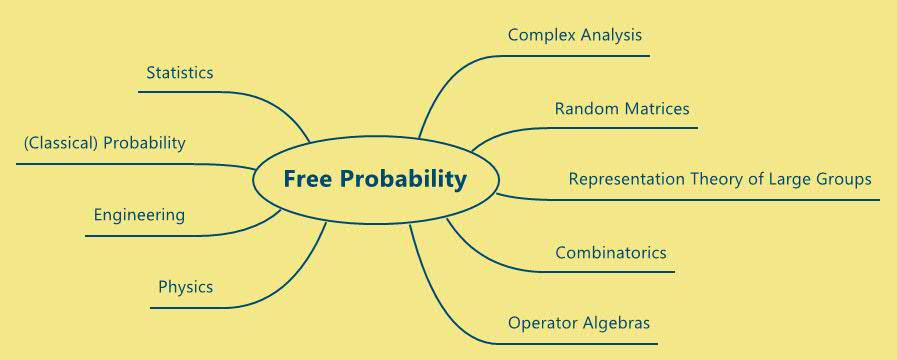## What is Free Probability?### A motivating example

Consider the following three natural questions from the fields of operator algebras,
random matrices, and combinatorics, respectively.
• What is the distribution of the real part of the one-sided shift with respect
to the canonical vacuum expectation state?
• What is the asymptotic eigenvalue distribution of a Gaussian random matrix
in the limit of large matrix size?
• In how many ways can one connect 2n points on a circle pairwisely by n
non-intersecting chords?
Surprisingly, all three questions have essentially the same answer: both question 1
and 2 yield the semicircular distribution, and the answer to question 3 is given by the
Catalan numbers which are actually the even moments of the semicircular distribution.
This coincidence is not just superficial - a conceptual reason is given by Voiculescu's
free probability theory and its basic concept of freeness.

### Origins and aspects of free probability

Free probability theory originated from questions about the structure of von Neumann
algebras related to free groups, namely those related to constructions around free products
of groups. One of the big unsolved problems in operator algebra is the question, whether
the free group factors are all isomorphic or not. Free probability theory is a very promising
attempt to attack this problem.

But also, free probability theory has evolved into a field with links to many other
quite unrelated fields: most notably random matrices, but also combinatorics, representation
theory of large groups, mathematical physics, or as applied fields as financial correlations
and wireless communications. Roland Speicher developed a combinatorial approach to free
probability theory - which explains why the third of the above questions is related to the
other two - and this has played a major role in many of those investigations.

### Non-commutative distributions in free probability

Free probability theory deals with distributions of variables. Whereas in the above mentioned
example of the semicircular distribution one has only one variable, the main interest is actually
the multivariate situation, where one has several variables. Typically those variables do not
commute, so one wants to understand non-commutative distributions. Such distributions may
arise in the large N limit of random multi-matrix models, as the distribution of operators in
operator algebras, or they might encode interesting combinatorial information. In each of those
fields the understanding of properties of the non-commutative distribution is related to
fundamental problems.
• In random matrix theory: to the existence and properties of the limit of multi-matrix
models;
• In operator algebras: to one of the most famous open problems, namely the isomorphism
problem for free group factors; and more generally, to invariants for von Neumann algebras;
• In combinatorics: to the counting of planar graphs or maps.
One should note that for one variable or, more general, for several commuting variables one has
very powerful classical analytical tools (like Fourier transform) for dealing with those objects. In
the non-commutative, however, all those classical tools break down and one is in need of a new
kind of non-commutative analysis (aka free analysis). Such a theory is still in its infancy.

In the projects of the ERC Advanced Grant NCDFP, held by Roland Speicher, the aim is to develop
the tools for dealing with such non-commutative distributions.

### Links to web pages concerning free probability

Workshop 2004 on free probability theory, Banff (including Final Report)
Workshop 2008 on free probability, extensions and applications, Banff (including Final Report)
Workshop on free probability theory 2005, Oberwolfach (including Final Report)

Videos from the Focus Program on Noncommutative Distributions in Free Probability Theory
at the Fields Institute, Toronto, Canada (talks and expository lectures):
First Workshop, Inter-Program Workshop, Second Workshop

Survey articles by Roland Speicher
What is a free cumulant? (by Jonathan Novak and Piotr Sniady)
Voiculescu receives NAS award in mathematics
Conferences concerning free probability theory
Victor Perez Abreu's homepage about free probability (in Spanish)

Free probability theory on wikipedia (English)
Freie Wahrscheinlichkeitstheorie auf Wikipedia (German)

 updated: 30 September 2014   Moritz Weber Impressum